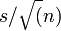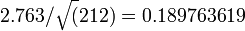# Standard Error

The standard deviation of the sampling distribution of a statistic. 

The standard error is a measure of precision - estimates with higher standard errors have lower precision - and is used in the computation of confidence intervals and many tests of statistical significance.

## Computation

There are a number of common methods of computing standard errors:

• Formulas for computing the standard errors directly from data. For example, the most well known computation of the standard error is the standard error of the mean which is computed as$s / \sqrt(n)$, were s is the Standard Deviation and n is the sample size.
• Formulas for computing the standard errors from regression outputs (sometimes referred to as analytic standard errors).
• Algorithms for approximating the hessian, which is then used as an input into formulas for computing standard errors (sometimes referred to as numeric standard errors). This is generally the method employed with Mixed Multinomial Logit Model. It is commonly employed when analytic standard errors cannot be computed.
• Resampling methods, including bootstrapping, jackknifes and permutations'. These are commonly used when it is believed the assumptions for the above three methods are not met.
• Using intermediate calculations from Bayesian estimation methods (i.e., the posterior distributions of the parameters).

Where the assumptions of each of the methods are met, they all compute the same standard error.[note 1] The only exception to this is Bayesian estimation methods, which often have slightly different goals and thus can lead to different results.

## Example

If the standard deviation of a variable in a Simple Random Sample is 2.763 and the sample size is 212 then the standard error is:$2.763 / \sqrt(212 ) = 0.189763619$

See Confidence Interval for the use of this calculation.